# F. Variational Derivation of Self-Energies

We consider the time evolution of the GREEN's function under the action of the time-independent HAMILTONian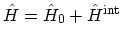and the time-dependent external perturbation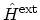. The latter is included through the evolution operator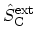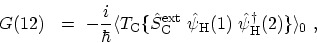(F.1)

where the abbreviationis used. To obtain the equation of motion, one can take the derivative of the GREEN's function with respect to time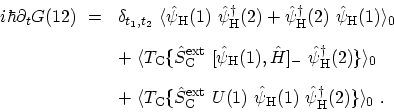(F.2)

The first contribution results from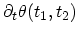(see Section 3.7.1). Because of the anti-commutation relation of the field operators it can be reduced to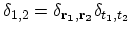. The equation of motion for the field operator,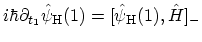, has been used in the second term, and the third contribution results from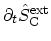. Inserting the commutator with the HAMILTONian, one obtains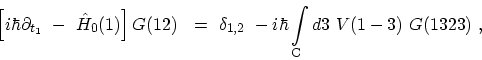(F.3)

where the two-particle GREEN's function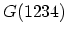is defined by(F.4)

To evaluate the two-particle GREEN's functions, one can express it as products of single-particle GREEN's functions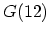, yielding an infinite perturbation expansion [203,282,205]. This can be accomplished by utilizing the GREEN's functions as generating functional. The two-particle GREEN's function can be expressed by means of functional derivatives of the single-particle GREEN's functions with respect to the external potential. Based on the variational method, the electron-electron and electron-phonon self-energies are derived next.
Subsections M. Pourfath: Numerical Study of Quantum Transport in Carbon Nanotube-Based Transistors